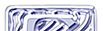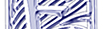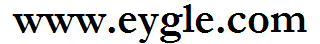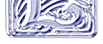首页 技术基础 备份恢复 SQL优化 诊断案例 BLOG 留言板 服务 生活 网摘 阅读 下载 智能巡检 English

### 使用Merge Into 语句实现 Insert/Updateif exists(select 1 from T where T.a='1001' ) update T set T.b=2 Where T.a='1001' else insert into T(a,b) values('1001',2);MERGE INTO table_name alias1USING (
table|view|sub_query) alias2ON (join condition)WHEN MATCHED THENUPDATE table_nameSET col1 = col_val1,col2
= col2_valWHEN NOT MATCHED THENINSERT (column_list) VALUES (column_values);MERGE INTO T T1USING (
SELECT a,b FROM T WHERE t.a='1001') T2ON ( T1.a=T2.a)WHEN MATCHED THENUPDATE SET T1.b = 2WHEN NOT MATCHED THENINSERT (a,b) VALUES('1001',2);MERGE INTO T T1USING (
SELECT '1001' AS a,2 AS b FROM dual) T2ON ( T1.a=T2.a)WHEN MATCHED THENUPDATE SET T1.b = T2.bWHEN NOT MATCHED THENINSERT (a,b) VALUES(T2.a,T2.b);MERGE INTO T T1USING (
SELECT Count(*) cnt FROM T WHERE T.a='1001') T2ON (T2.cnt>0)WHEN MATCHED THENUPDATE SET T1.b = T2.bWHEN NOT MATCHED THENINSERT (a,b) VALUES(T2.a,T2.b);

By eygle on 2009-01-22 21:45 | Comments (2) | Oracle摘 | 2236 |